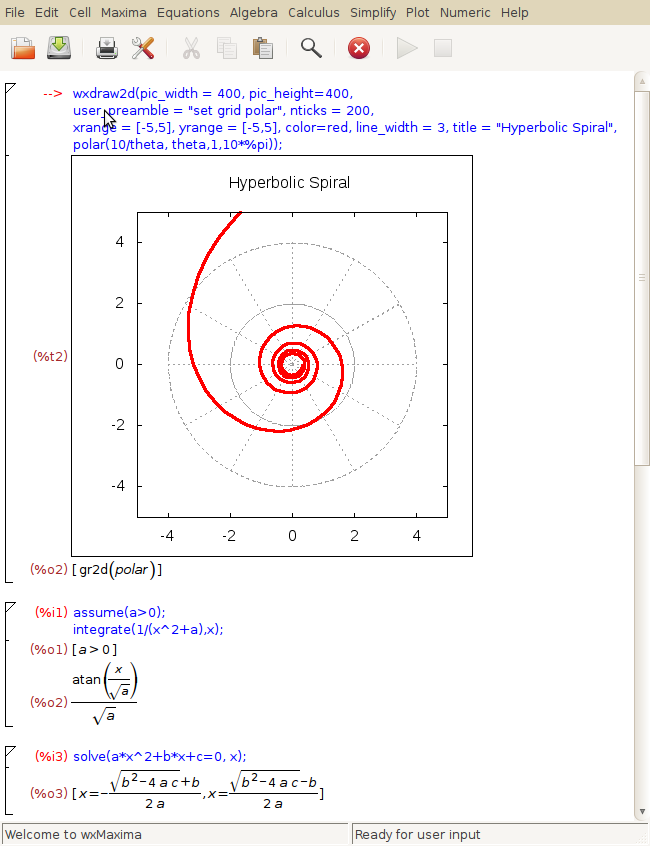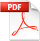# Introductory Computer Algebra

## OverviewSample wxMaxima notebook.
The Department wants students to work towards being independent learners. As part of the redesign of the undergraduate programme, it has therefore incorporated the use of computer algebra in its teaching.
Computer algebra software can help you learning and doing mathematics. It may be used to reduce the tedium of extended calculations, to verify the correctness of hand calculation and also for exploration of a new topic. It is not a substitute for mathematical thinking. Think about it: if a computer program could solve all mathematical problems, there would be no jobs for people with maths degrees. Note that computer algebra software usually, but not always, gets the right answer.
Computer algebra is embedded in many modules and is used by lots of lecturers for demonstrations and to ease calculations. All students studying a Mathematics degree have to do a project in their final year, and by learning how to use computer algebra at an early stage you are more likely to fix concepts practically and benefit from what it has to offer before you get to your final year.

## Structure of this module

There will be an initial supervised/guided session at the beginning of the year, where students will go through a worksheet showing how computer algebra may be used in the context of some A-level topics. During the remainder of the year, from time to time lecturers will demonstrate ways in which computer algebra may be used to check calculations, carry out more difficult computations and gain insight into the material being studied. Lecturers may also set problems to be solved by students using computer algebra.

## Guides and Lecture notesCIS Guide 65 - Basic Maple: a Beginner's Guide This is the CIS beginner's guide to Maple with a brief overview of how the system works.Introduction to Computer Algebra Notes to get you started, using Maple to illustrate the type of problems you can tackle with computer algebra.
Finally, the best thing you can do to learn a computer algebra system is to simply experiment with one of them.

## Getting computer algebra software

There are several general purpose computer algebra systems available, some for free (e.g. Maxima and the graphical version wxMaxima) and others for a substantial price (e.g. Mathematica). At Durham University we have a site licence for Maple, which is available to use on all IT service networked computers; for more information see the CIS Maple page. Once registered and in possession of a campus card, students who wish to purchase Maple for their own computers can do so from the CIS service desk for the substantially discounted price of £15.
These "three M's" can all handle algebra, calculus, linear algebra, plotting and so on, and have a varying degree of built-in knowledge about special functions, differential equations and other more advanced topics. So they will most likely get you through a lot of material of the first few years of a maths degree. However, sometimes you may run into a problem for which none of these general purpose tools are any good; feel free to contact me if you want to know more about other options.

## Sample notebooks/worksheets

notebookMapleMathematicawxMaxima
tutorial tutorial.mw tutorial.nb tutorial.wxm

## Which Durham modules use computer algebra?

In Mathematical Sciences teaching we use Maple in a number of modules, listed below. Some modules also use MapleTA through DUO.
 Core A MATH1012 Data analysis, modelling & simulation MATH1711 Single mathematics A MATHS1561 Core Mathematics B2 MATHS1041 Numerical Analysis II MATHS2051 Differential Geometry III MATHS3021 Approximation Theory & Solutions of ODEs III MATHS3081 Electromagnetism III MATHS3181 Solitons III MATHS3231 Solitons IV MATHS4121 Approximation Theory & Solutions of ODEs IV MATHS4221